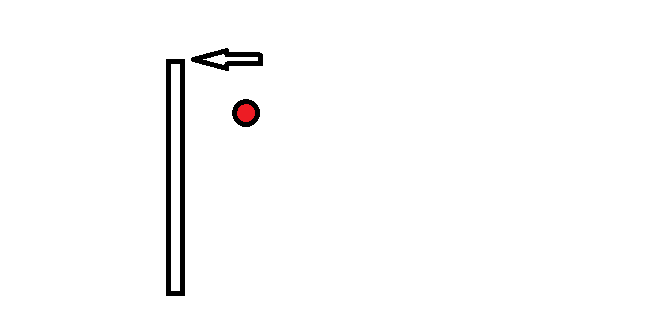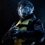# Scope of coefficient of restitutionThere are several several instances when we can use the fact that $e=1$. to simplify collision problems which saves us from having to apply conservation of energy.

The result $e=1$. is fairly obvious for head-on collisions, and for collisions of extended objects involving pure translation and for those involving pure rotation.

But my doubt is can I in general use the result for any collision among extended bodies too which might involve a combination of both rotation and translation ??

Can I say that if an object elastically collides with another,,, then the velocities of the points of contacts of both objects satisfy the relation $e=1$. ??

example in the image,, if the collision between ball and rod is elastic,, can i say that the point of collision of the rod after collision necessarily moves at a speed v such that,, if the balls initial and final velocities are $u$. and $f$. respectively ,

then ,

$Tell$ $me$ whether I use :

1) $v-f=u$. ??

or

2) $e=1$ ??

or

3) Do I have to use the usual way of energy conservation and momentum conservation and angular momentum conservation independently ??

Please any one who has the knowledge I seek help me ,, if possible also provide a qualitative or quantitative explanation

$Be$ $Careful$. :

e is the coefficient of restitution, not to be confused with $2.7108$.

$NOTE$. :

I know it is applicable for pure rotating and pure translating objects whether extended or not because i can prove it)Note by Mvs Saketh
6 years, 9 months ago

This discussion board is a place to discuss our Daily Challenges and the math and science related to those challenges. Explanations are more than just a solution — they should explain the steps and thinking strategies that you used to obtain the solution. Comments should further the discussion of math and science.

When posting on Brilliant:

• Use the emojis to react to an explanation, whether you're congratulating a job well done , or just really confused .
• Ask specific questions about the challenge or the steps in somebody's explanation. Well-posed questions can add a lot to the discussion, but posting "I don't understand!" doesn't help anyone.
• Try to contribute something new to the discussion, whether it is an extension, generalization or other idea related to the challenge.

MarkdownAppears as
*italics* or _italics_ italics
**bold** or __bold__ bold
- bulleted- list
• bulleted
• list
1. numbered2. list
1. numbered
2. list
Note: you must add a full line of space before and after lists for them to show up correctly
paragraph 1paragraph 2

paragraph 1

paragraph 2

[example link](https://brilliant.org)example link
> This is a quote
This is a quote
    # I indented these lines
# 4 spaces, and now they show
# up as a code block.

print "hello world"
# I indented these lines
# 4 spaces, and now they show
# up as a code block.

print "hello world"
MathAppears as
Remember to wrap math in $$ ... $$ or $ ... $ to ensure proper formatting.
2 \times 3 $2 \times 3$
2^{34} $2^{34}$
a_{i-1} $a_{i-1}$
\frac{2}{3} $\frac{2}{3}$
\sqrt{2} $\sqrt{2}$
\sum_{i=1}^3 $\sum_{i=1}^3$
\sin \theta $\sin \theta$
\boxed{123} $\boxed{123}$

Sort by:

My question Challenges in mechanics by Ronak Agarwal (Part3) is based on this concept only.

- 6 years, 9 months ago

gotta try it then,, i saw it before but it seemed to lengthy,, either way can you prove that e=1 for all sorts of elastic collisions? or is it just a general result you know and apply ? :)

- 6 years, 9 months ago

Yes it is a general result that $e=1 \Rightarrow$ Energy is conserved.

- 6 years, 9 months ago

got it,, and yes i got the correct solution to ur cricket answer,,, but why i cant i see the add answer button anymore,, either way applying conservation of angular momentum gives the same equation structure as my previous admittedly wrong manner,, what we need is that after the collision the velocity of ball should be directed towards a wicket,,, but the direction of angular momentum cannot be changed and hence the angle between the initial angular momenta by virtue of rotation and translation should be such that they match with the angle of line joining the extreme wicket and the line representing perpendicular distance from the wickets,, correct?

- 6 years, 9 months ago

Now you are exactly right.

- 6 years, 9 months ago

Yes you can in general use the result e=1 for any kind of collisions.

- 6 years, 9 months ago

Why do you put two commas ,, where ever you have used them ?

- 6 years, 9 months ago

I had edited Them For $Saketh$.

- 6 years, 9 months ago